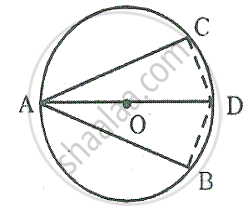Share

In the Following Figure, Ab is the Diameter of a Circle with Centre O. If Chord Ac = Chord Ad, Prove That: (I) Arc Bc = Arc Db (Ii) Ab is Bisector of ∠Cad. Further, If the Length of Arc Ac is Twice - ICSE Class 10 - Mathematics

ConceptArc and Chord Properties - Angle in a Semi-circle is a Right Angle

Question

In the following figure, AB is the diameter of a circle with centre O.
If chord AC = chord AD, Prove that:
(i) arc BC = arc DB
(ii) AB is bisector of ∠CAD.
Further, if the length of arc AC is twice the length of arc BC, find : (a) ∠BAC (b) ∠ABC

SolutionGiven – In a circle with centre O, AB is the diameter and AC and AD are two chords such that AC = AD
To prove: (i) arc BC = arc DB
(ii) AB is the bisector of ∠CAD
(iii) If arc AC = 2 arc BC, then find

(a) ∠BAC

(b) ∠ABC

Construction: Join BC and BD
Proof : In right angled ∆ABC and ∆ABD Side AC = AD          [Given]
Hyp. AB = AB   [ Common]
∴ By right Angle – Hypotenuse – Side criterion of congruence

ΔABC ≅ ΔABD

(i) The corresponding parts of the congruent triangle are congruent.
∴ BC = BD     [c.p.c.t]
∴ Arc BC = Arc BD [ equal chords have equal arcs]

(ii) ∠BAC = ∠BAD
∴ AB is the bisector of ∠CAD

(iii) If Arc AC = 2 arc BC,
Then ∠ABC = 2∠BAC
But ∠ABC + ∠BAC = 90°
⇒2∠BAC + ∠BAC = 90°
⇒ 3∠BAC = 90°

⇒ ∠BAC = (90°)/3= 30°
∠ABC = 2∠BAC ⇒ ∠ABC = 2 ×30° = 60°

Is there an error in this question or solution?

Video TutorialsVIEW ALL 

Solution In the Following Figure, Ab is the Diameter of a Circle with Centre O. If Chord Ac = Chord Ad, Prove That: (I) Arc Bc = Arc Db (Ii) Ab is Bisector of ∠Cad. Further, If the Length of Arc Ac is Twice Concept: Arc and Chord Properties - Angle in a Semi-circle is a Right Angle.
S